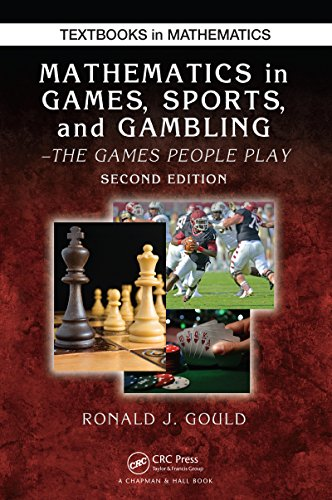Gambling Portal

# Mathematics in Games, Sports, and Gambling: The Games People Play, Second Edition (Textbooks in Mathematics)Price:Mathematics in Games, Sports, and Gambling: The Games People Play, Second Edition demonstrates how discrete probability, statistics, and elementary discrete mathematics are used in games, sports, and gambling situations. With emphasis on mathematical thinking and problem solving, the text draws on numerous examples, questions, and problems to explain the application of mathematical theory to various real-life games.

This updated edition of a widely adopted textbook considers a number of popular games and diversions that are mathematically based or can be studied from a mathematical perspective. Requiring only high school algebra, the book is suitable for use as a textbook in seminars, general education courses, or as a supplement in introductory probability courses.

New in this Edition:

• Many new exercises, including basic skills exercises

• More answers in the back of the book

• Expanded summary exercises, including writing exercises

• More detailed examples, especially in the early chapters

• An expansion of the discrete adjustment technique for binomial approximation problems

• New sections on chessboard puzzles that encourage students to develop graph theory ideas

• New review material on relations and functions

Exercises are included in each section to help students understand the various concepts. The text covers permutations in the two-deck matching game so derangements can be counted. It introduces graphs to find matches when looking at extensions of the five-card trick and studies lexicographic orderings and ideas of encoding for card tricks.

The text also explores linear and weighted equations in the section on the NFL passer rating formula and presents graphing to show how data can be compared or displayed. For each topic, the author includes exercises based on real games and actual sports data.